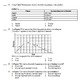# Physical Science: Unit 7 Physics Forces and MotionSubject
Resource Type
File Type
Zip (21 MB|100+)
\$5.60
List Price:
\$9.00
Bundle Price:
\$7.00
You Save:
\$3.40
\$5.60
List Price:
\$9.00
Bundle Price:
\$7.00
You Save:
\$3.40
Product Description
How to get TPT credit to use on future purchases:
• Please go to your My Purchases page (you may need to login). Beside each purchase you'll see a Provide Feedback button. Simply click it and you will be taken to a page where you can give a quick rating and leave a short comment for the product

Unit Title: Physics Forces and Motion

Included
6 - PowerPoint Presentations (pptx) 62 slides
18 - Worksheets/ Labs (docx)
4 - Unit Tests (CP and Pre-AP) with answer key (Examview and docx)
3 - Unit Reviews and Test Guides with Vocabulary (docx)

Subject/Topic Areas:
Measuring Motion (speed & velocity), Acceleration & Motion (calculating and graphing acceleration), Fundamental Forces, Balanced & Unbalanced Forces, Newton’s 3 Laws of Motion, Weight & Mass, Law of Universal Gravitation, Free- Fall Acceleration, Projectile Motion

Brief Summary of Unit

Enduring Understanding:
Students will understand that:
• Newton’s three laws of motion explain the motion of all objects.
• The gravitational force between two objects is related to the distance and mass of the objects.
Essential Question:
• What is the difference between velocity and acceleration?
• What is the difference between mass and weight?
• How do Newton’s Laws affect all motion on Earth?
• How is momentum related to mass and velocity?
• What is the Law of Conservation of Momentum?

Students will know: (Knowledge)
• To calculate the acceleration of an object, the change in velocity or speed is divided by the length of time interval over which the change occurred.
• Newton’s 1st Law of Motion states that an object moving at a constant velocity keeps moving at that velocity unless a net force acts on it.
• Newton’s 2nd Law of Motion states the net force acting on an object causes the object to accelerate in the direction of the net force.
• Newton’s 3rd Law of Motion states for every action force there is an equal and opposite reaction force.
• Gravity and friction affect all motion on Earth.
Students will be able to do (Skills)
• Calculate velocity and acceleration.
• Apply Newton’s three laws to real world situations by explaining the following: inertia, relationship between force, mass, and acceleration, equal and opposite forces
• Calculate force.
• Identify the different types of friction and its effect on motion.

Learning Activities
• Cornell notes
• Collaborative groups
• Distributed practice (speed, velocity, acceleration, weight and momentum problems)
• Discovery or Inquiry learning (Speed Challenge Activity, Acceleration Lab)
• Experiment
• Compare and Contrast (Newton’s 3 Laws of Motion, Static & Kinetic Friction)
• Anticipation activity (graphic organizer)
• Vocabulary

Differentiation:
• Pair students; students with high ability, low ability, and other.
• Extended time on tests or assignments
• Enhanced learning material

Relevant Content Vocabulary

Motion, frame of reference, displacement, speed, velocity, acceleration, force, friction, static friction, kinetic friction
Inertia, weight, free-fall acceleration, terminal velocity, projectile motion, momentum
Total Pages
100+